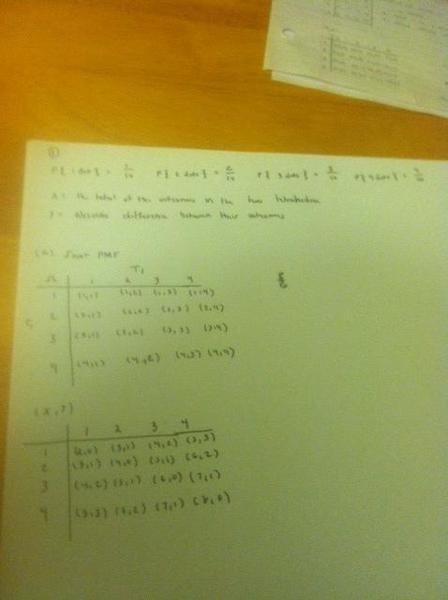# Discrete Bivariate Random Variables

Here is the question:

Consider the experiment of rolling two tetrahedra that are unfair in the sense that each has the following probabilities for each of the four faces:

P{1 dot}=1/10
P{2 dots}=2/10
P{3 dots}=3/10
P{4 dots}=4/10

Let X be the total of the outcomes in the two tetrahedra and Y be the absolute difference between their outcomes. Obtain the joint probability mass function of (X,Y).

So I am confused on how to do the probabilities since it is unfair and not fair. Help.

Here is the work I've done, I'm stuck and not really sure how to transform this into the joint probability mass function for (X,Y).Sorry the photo's sideways, I tried rotating it but it wouldn't translate it into the post.

## Answers and Replies

Homework Helper
Dearly Missed
Here is the question:

Consider the experiment of rolling two tetrahedra that are unfair in the sense that each has the following probabilities for each of the four faces:

P{1 dot}=1/10
P{2 dots}=2/10
P{3 dots}=3/10
P{4 dots}=4/10

Let X be the total of the outcomes in the two tetrahedra and Y be the absolute difference between their outcomes. Obtain the joint probability mass function of (X,Y).

So I am confused on how to do the probabilities since it is unfair and not fair. Help.

Here is the work I've done, I'm stuck and not really sure how to transform this into the joint probability mass function for (X,Y).Sorry the photo's sideways, I tried rotating it but it wouldn't translate it into the post.

## The Attempt at a Solution

Are the two tetrahedra independent? If so, and if X1 = value on tet. 1 and X2 = value on tet. 2, then X1 and X2 are independent and have known probability distributions. So, in this case what is the bivariate distribution of the pair (X1,X2)? [Maybe your attached work has already done this, but I can't read it.] Now just get X and Y in terms of X1 and X2.

RGV